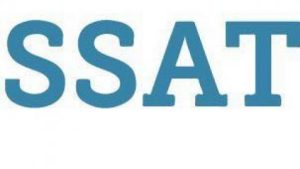# Do You Know How to Interpret Your Child’s SSAT Score?

## SSAT Score and Score Reporting

Your child’s SSAT score report will contain the following information:

• Number of Items: The number of items in the content sections and subsections.
• Number Correct: The number of correct answers for the content sections and subsections.
• Percent Correct: The percentage of correct answers for the content sections and subsections.
• Scaled Score: A score which has a range of values from 300 to 600. The mean value of the content sections’ scaled scores is 450.
• Scaled Score Percentile Rank: The scaled score percentile rank is a score which has values from 1 to 99. It compares performance to other students taking the same examination.
• Total Scaled Score: The total scaled score is the sum of the scaled scores for the quantitative, verbal, and reading sections. It has a low value of 900, a high value of 1800, and a mean of 1350.

## What You’ll See:

### The Test You Took

You took the Middle Level SSAT if you’re in grades 5-7 and the Upper Level SSAT if you’re in grades 8-11. There is a different score scale for each level.

This section contains your personal information. Be sure that your name and date of birth are correct. Also check to ensure that you registered using your current grade, which determines which test level you took and the comparison/norm group for the SSAT percentiles.

SSAT scoring is broken down by section (verbal, quantitative/math, reading). A total score (a sum of the three sections) is also reported.

For the Middle Level SSAT, the lowest number on the scale (440) is the lowest possible score a student can earn and the highest number (710) is the highest score for each section.The total scaled score for a Middle Level SSAT is the sum of the scores for the quantitative, verbal, and reading sections. It has a low value of 1320, a high value of 2130, and a midpoint of 1725.

For the Upper Level SSAT, the lowest number on the scale (500) is the lowest possible score a student can earn and the highest number (800) is the highest score for each section. The total scaled score for an Upper Level
SSAT is the sum of the scores for the quantitative, verbal, and reading sections. It has a low value of 1500, a high value of 2400, and a midpoint of 1950.

### SSAT Reference Information

The SSAT Percentile (1 to 99) compares your performance on the SSAT with that of other students of the same grade/gender who have taken the SSAT in the U.S. and Canada on a Standard test date in the previous three years (the norm group). For students who have taken the SSAT more than once, only their first set of scores is included in this process. You will see the average scoring for the norm group for each section of the SSAT, as well as your percentile as compared to the norm group.

### Scaled Scoring Range

No single test score provides a perfectly accurate estimate of your proficiency.  Therefore, a score range is provided to emphasize the possiblity of score differences if you took the SSAT again within a relatively short period of time. Your new scores would likely fall within the scaled score range indicated.

### Test Question Breakdown

This section provides valuable information about the test’s content and your test-taking strategies. Comparing the number of wrong answers to unanswered questions will tell you whether you took many guesses rather than leaving questions unanswered if you weren’t sure of the answer.